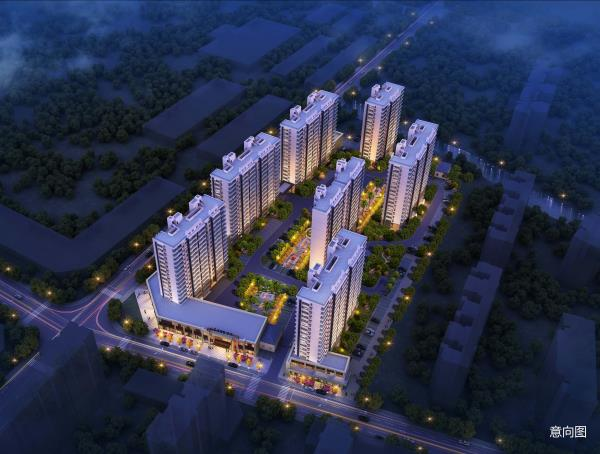## 网站地图## 碧桂园·繁华里

### 嘉兴

#### 地址

• 资阳
• 本溪
• 北流
• 万载
• 慈溪
• 长春
• 通化
• 西昌
• 丰城
• 阳春
• 鹤山
• 江油
• 辛集
• 辽源
• 衡水
• 黔西南州
• 黔东南州
• 石家庄
• 唐山
• 秦皇岛
• 邯郸
• 邢台
• 保定
• 张家口
• 承德
• 沧州
• 廊坊
• 黄骅
• 迁安
• 怀来
• 洛阳
• 驻马店
• 郑州
• 开封
• 平顶山
• 安阳
• 鹤壁
• 新乡
• 焦作
• 濮阳
• 许昌
• 漯河
• 三门峡
• 南阳
• 商丘
• 信阳
• 周口
• 武汉
• 黄石
• 十堰
• 宜昌
• 襄阳
• 荆州
• 荆门
• 鄂州
• 仙桃
• 潜江
• 黄冈
• 随州
• 咸宁
• 恩施
• 怀化
• 长沙
• 株洲
• 湘潭
• 湘西州
• 张家界
• 益阳
• 郴州
• 衡阳
• 岳阳
• 常德
• 娄底
• 徐州
• 南京
• 无锡
• 镇江
• 泰州
• 淮安
• 舟山
• 黔西南布依族苗族自治州
• 临沂
• 凉山
• 普洱
• 红河
• 锦州
• 葫芦岛
• 东方
• 咸阳
• 丽水
• 定安县
• 吕梁
• 来宾
• 河池
• 宜宾
• 重庆市
• 雅安
• 潮州
• 大理
• 巴中
• 桂林
• 贵港
• 百色
• 柳州
• 贺州
• 儋州
• 福清
• 威海
• 烟台
• 乐山
• 金华
• 永泰
• 潍坊
• 滨州
• 德州
• 临汾
• 日照
• 眉山
• 武威
• 乌鲁木齐
• 保山
• 临沧
• 防城港
• 达州
• 遂宁
• 绵阳
• 淮南
• 宝鸡
• 枣庄
• 桂平
• 景德镇
• 临夏
• 聊城
• 崇左
• 马鞍山
• 安顺
• 北海
• 菏泽
• 铜陵
• 黔南州
• 蚌埠
• 嘉兴
• 淮北
• 都匀
• 南宁
• 兴义
• 南平
• 松溪
• 吉安
• 大同
• 陆丰
• 厦门
• 铜仁
• 银川
• 昭通
• 包头
• 遵化
• 漳州
• 深圳
• 霸州
• 香港
• 合肥
• 抚州
• 琼海
• 定西
• 南昌
• 宿州
• 文昌
• 临高县
• 陵水
• 海口
• 博鳌
• 三亚
• 绥化
• 哈尔滨
• 杭州
• 绍兴
• 宁波
• 温州
• 衢州
• 湖州
• 台州
• 天津
• 沈阳
• 大连
• 通辽
• 兴安盟
• 呼伦贝尔
• 运城
• 晋中
• 太原
• 南通
• 宿迁
• 扬州
• 盐城
• 连云港
• 苏州
• 常州
• 安庆
• 黄山
• 巢湖
• 芜湖
• 池州
• 宣城
• 六安
• 古田
• 无为
• 桐城
• 亳州
• 南陵
• 庐江
• 阜阳
• 滁州
• 孝感
• 天门
• 邵阳
• 永州
• 平果
• 玉林
• 梧州
• 钦州
• 梅州
• 河源
• 云浮
• 东莞
• 汕头
• 中山
• 清远
• 惠州
• 韶关
• 肇庆
• 阳江
• 汕尾
• 茂名
• 江门
• 佛山
• 广州
• 雷州
• 英德
• 湛江
• 揭阳
• 珠海
• 信宜
• 济南
• 淄博
• 青岛
• 泰安
• 济宁
• 马来西亚雪兰莪州
• 马来西亚柔佛州
• 永春
• 南安
• 三明
• 宁德
• 武夷山
• 福鼎
• 莆田
• 建瓯
• 漳浦
• 福安
• 福州
• 泉州
• 龙岩
• 兰州
• 张掖
• 天水
• 白银
• 贵阳
• 仁怀
• 毕节
• 凯里
• 六盘水
• 遵义
• 广元
• 德阳
• 南充
• 泸州
• 攀枝花
• 广安
• 成都
• 宜春
• 萍乡
• 九江
• 赣州
• 上饶
• 曲靖
• 楚雄
• 昆明
• 白城
• 北京
• 澳洲莱德
• 西安
• 渭南
• 上海
• 西宁毗邻振东新区 时尚产业中心

项目位于沪嘉杭G60科创走廊交汇处，毗邻振东新区，地处中国首批特色小镇——濮院镇重要地带。距离濮院羊毛衫市场仅约3公里，繁华所向，蓄势待发。

以上数据来源于百度地图

立体交通 链接沪杭都市圈

项目位于桐乡濮院镇，北临紫金路，南接凯旋路，经濮院大道(G320国道)可直达桐乡市区、嘉兴等地。桐乡高铁站、申嘉湖高速和G60沪昆高速，立体交通畅享沪杭都市生活圈。

以上数据来源于百度地图

商圈汇聚 纵享繁华

项目毗邻佳源广场、濮院中心集贸市场，距离约2公里内有桐乡第四人民医院、幽湖公园、濮院中心广场，医疗、购物、休闲娱乐，一应俱全。

以上数据来源于百度地图

学府书香 助力孩子美好未来

濮院文教新区旁，约500米内即达濮院新区幼儿园、濮院新星学校、濮院镇中心幼儿园，约2公里内可达濮院茅盾实验小学、翔云小学和濮院第三中学，优质教育链，助力孩子美好未来。

以上周边教育配套设施，不作为学区承诺，具体入学条件以政府相关文件为准。

精工匠筑 美好生活

碧桂园，以1200多个城镇、超400万业主的居住体验为研究样本，为濮院人民量身打造建筑面积约96—135㎡好房子系列产品，缔造2019濮院人居时尚新体验。

A户型，建筑面积约96㎡，三室两厅两卫

B户型，建筑面积约106㎡，三室两厅两卫

C户型，建筑面积约135㎡，四室两厅两卫项目推广名：“碧桂园·繁华里”，核准地名“繁华里”。本资料仅为要约邀请，不作为出卖人向买受人发出之购房要约，本资料中仅是表述碧桂园·繁华里项目总体布局建筑物的大致方位，楼栋、道路、园林绿化、空间距离、配套设施等仅为效果示意，与实际存有差异;资料中的图文、数据信息等仅供参阅。具体规划方案以政府批准、买卖双方签署的《商品房买卖合同》等法律文书以及现场实际交付的为准。本资料中描述本项目周边环境、交通、公共设施等地理关系示意，是按制作本图纸时政府已批的规划、现状及出卖人收集的信息绘制而成，旨在提供相关信息供参阅，非出卖人之具体承诺;也并不排除政府相关部门或第三方会对相关功能设施设备做相应的调整。本资料中建筑物区位、路线等已经简化处理，不代表实际距离比例，所有路线仅供参阅之用。相关内容如有更新，请以最近资料为准，敬请留意。本资料绘制日期：2019年7月1日。